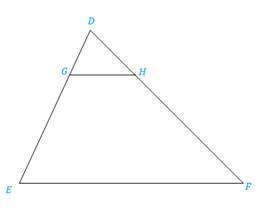Chapter 5.3, Problem 22EElementary Geometry For College St...

7th Edition
Alexander + 2 others
ISBN: 9781337614085

Solutions

Chapter
SectionElementary Geometry For College St...

7th Edition
Alexander + 2 others
ISBN: 9781337614085
Textbook Problem

In Exercises 17 to 24, complete each proof.Given: D G D E = D H D F Prove: ∠ D G H ≅ ∠ EPROOF Statements Reasons 1. ?2. ∠ D ≅ ∠ D 3. Δ D G H ∼ Δ D E F 4. ? 1. ?2. ?3. ?4. ?

To determine

To prove:

The statement DGHE if the proportions are given DGDE=DHDF.

Explanation

Definition:

SAS:

If an angle of one triangle is congruent to an angle of a second triangle and the pairs of sides including the angles are proportional (in length), then the triangles are similar.

CASTC:

Corresponding angles of similar triangles are congruent.

Description:

Given that DGDE=DHDF.

The given figure is shown below.

Figure

From the given figure, it is observed that DGDE=DHDF are the pairs of corresponding sides which are proportional and also noticed that the common point of both triangles is D.

Hence, by identity DD.

The above mentioned SAS definition, the two triangles DGH and DEF are similar since an angle of one triangle is congruent to an angle of a second triangle and the pairs of sides including the angles are proportional (in length)

Still sussing out bartleby?

Check out a sample textbook solution.

See a sample solution

The Solution to Your Study Problems

Bartleby provides explanations to thousands of textbook problems written by our experts, many with advanced degrees!

Get Started

Convert the expressions in Exercises 8596 radical form. 94(1x)7/3

Finite Mathematics and Applied Calculus (MindTap Course List)

Write the following numbers in word form. 2.48

Contemporary Mathematics for Business & Consumers

Which is the best graph of y = 1.5x? (The graph of y = 2x is shown as a dashed curve for comparison.)

Study Guide for Stewart's Single Variable Calculus: Early Transcendentals, 8th

It does not exist.

Study Guide for Stewart's Multivariable Calculus, 8th

Identify possible sources and use them to identify a topic area for research.

Research Methods for the Behavioral Sciences (MindTap Course List)